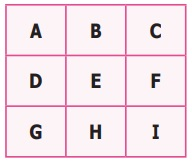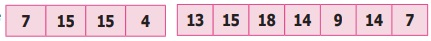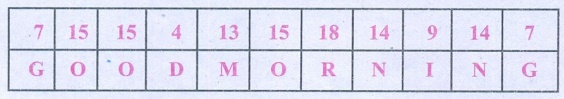Home | | Maths 6th Std | Exercise 5.2

# Exercise 5.2

6th Maths : Term 3 Unit 5 : Information Processing : Exercise 5.2: Miscellaneous Practice problems, Challenge problems , Text Book Back Exercises Questions with Answers, Solution

Exercise 5.2

Miscellaneous Practice problems

1. Find HCF of 188 and 230 by Euclid's game.

H.C.F. of 188 and 230

188 = 2 ├Ś 2 ├Ś 47

230 = 2 ├Ś 5 ├Ś 23

H.C.F. of (188 , 230) = 2

H.C.F. of (188, 230 ŌłÆ 188)

188 = 2 ├Ś 2 ├Ś 47

42 = 2 ├Ś 3 ├Ś 7

H.C.F. of (188, 42)  = 2

H.C.F. of (188 , 230) =    H.C.F. of  (188, 230 ŌłÆ 188)

2. Write the numbers from 1 to 50. From that find the following.

1 2 3 4 5 6 7 8 9 10

11 12 13 14 15 16 17 18 19 20

21 22 23 24 25 26 27 28 29 30

31 32 33 34 35 36 37 38 39 40

41 42 43 44 45 46 47 48 49 50

i) The numbers which are neither divisible by 2 nor 7.

Answer: 9, 11, 13, 15, 17, 19, 23, 25, 27, 29, 31, 33, 37, 39, 41, 43, 45, 47.

ii) The prime numbers between 25 and 40.

iii) All square numbers upto 50.

Answer: 1, 4, 9, 16, 25, 36, 49

3. Complete the following pattern

i) 1+2+3+4 = 10

2+3+4+5 = 14+4+5+6 =4+5+6+=1 + 2 + 3 + 4 = 10

2 + 3 + 4 + 5 = 14

3 + 4 + 5 + 6 = 18

4 + 5 + 6 + 7 = 22

ii) 1+3+5+7 = 16+5+7+9 = 24

5+7+9+=7+9++13 =1 + 3 + 5 + 7 = 16

3 + 5 + 7 + 9 = 24

5 + 7 + 9 + 11 = 32

7 + 9 + 11 + 13 = 40

iii) AB, DEF, HIJK, ___ , STUVWX

AB, DEF, HIJK, MNOPQ , STUVWX

iv) 20, 19, 17,,10,5

20, 19, 17, 14 , 10, 5

4. Complete the table by using the following instructions.A: It is the 6th term in the Fibonacci sequence. 8

B: The predecessor of 2. 1

C: LCM of 2 and 3. 6

D: HCF of 6 and 20 2

E: The reciprocal of 1/5. 5

F: The opposite number of ŌĆō7.  7

G: The first composite number. 4

H: Area of a square of side 3 cm. 9

I: The number of lines of symmetry of an equilateral triangle. After completing the table, what do you observe? Discuss. 3After completing the table, what do you observe? Discuss.

5. Assign the number for English alphabets as 1 for A, 2 for B upto 26 for Z.

Find the meaning of6. Replace the letter by symbols as + for A, ŌĆō for B, ├Ś for C and ├Ę for D.

Find the answer for the pattern 4B3C5A30D2 by doing the given operations.

4 B 3 C 5 A 3 0 D 2

4 ŌĆō 3 ├Ś 5 + 30 ├Ę 2

4 ŌłÆ 15 + 15 = 4

7. Observe the pattern and find the word by hiding the numbers

1H2O3WŌĆā4A5R6EŌĆā7Y8O9U?

1H2O3W  4A5R6E  7Y8O9U?

HOW         ARE         YOU?

8. Arrange the following from the eldest to the youngest. What do you get?A ŌłÆ refers to parents

L ŌłÆ refers to you

F ŌłÆ refers to grandparents

I ŌłÆ refers to elder sister

Y ŌłÆ refers to younger brother

M ŌłÆ refers to uncle

FAMILY

Challenge problems

9. Prepare a daily time schedule for evening study at home.

5.30 p.m to 6.30 p.m ŌłÆ Writing Home work.

6.30 p.m to 7.30 p.m ŌłÆ Maths

7.30 p.m to 8.00 p.m ŌłÆ English

8.00 p.m to 9.00 p.m ŌłÆ Tamil, Science and Social science

9.00 p.m to 9.30 p.m ŌłÆ Drawing and extra work.

10. Observe the geometrical pattern and answer the following questionsi) Write down the number of sticks used in each of the iterative pattern.

ii) Draw the next figure in the pattern also find Name the total number sticks used in it.Number of sticks 30.

11. Find HCF of 28,35,42 by EuclidŌĆÖs game.

H.C.F. of  28, 35, 42.

28 = 2 ├Ś 2 ├Ś 7

35 = 5 ├Ś 7

42 = 2 ├Ś 3 ├Ś 7

H.C.F. of  (28, 35, 42) = 7

H.C.F. of  (28, 35 ŌłÆ 28, 42 ŌłÆ 28)

28 = 2 ├Ś 2 ├Ś 7

7 = 1 ├Ś 7

14 = 2 ├Ś 7

H.C.F. of  (28, 35 ŌłÆ 28, 42 ŌłÆ 28) = 7

H.C.F. of  (28, 35, 42) = H.C.F. of (28, 35 ŌłÆ28, 42 ŌłÆ28)

12. Follow the given instructions to fill your name in the OMR sheet.ŌĆó The name should be written in capital letters from left to right.

ŌĆó One alphabet is to be entered in each box.

ŌĆó If any empty boxes are there at the end they should be left blank.

ŌĆó Ball point pen is to be used for shading the bubbles for the corresponding alphabets.13. Consider the Postal Index Number (PIN) written on the letters as follows:604506; 604516; 604560; 604506; 604516; 604516; 604560; 604516; 604505; 604470; 604515; 604520;604303; 604509; 604470. How the letters can be sorted as per Postal Index Numbers?

604 is common for all postal index numbers. Compare the remaining 3 digits, 303, 470, 505, 506 (two), 509, 510, 515, 516 (four), 520, 560 (two).

Exercise 5.2

1. 2

2. i) 9, 11, 13, 15, 17, 19, 23, 25, 27, 29, 31, 33, 37, 39, 41, 43, 45, 47 ii) 29, 31, 37 iii) 1,4, 9, 16, 25, 36, 49

3. i) 3, 18; 7, 22 ii) 3; 11,32; 11, 40

iii) MNOPQ iv) 14

4. A-8, B-1, C-6, D-2, E-5, F-7, G-4, H-9, I-3

5. GOOD MORNING

6. 4

7. HOW ARE YOU?

8. FAMILY

10. i) 3, 9, 18 ii)30

11. 7

13. 604 is common for all postal index numbers. Compare the remaining 3 digits, 303, 470, 505, 506 (two), 509, 510, 515, 516 (four), 520, 560 (Two)

Tags : Questions with Answers, Solution | Information Processing | Term 3 Chapter 5 | 6th Maths , 6th Maths : Term 3 Unit 5 : Information Processing
Study Material, Lecturing Notes, Assignment, Reference, Wiki description explanation, brief detail
6th Maths : Term 3 Unit 5 : Information Processing : Exercise 5.2 | Questions with Answers, Solution | Information Processing | Term 3 Chapter 5 | 6th Maths